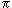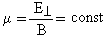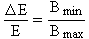# Measurement principle

Beta decay and MAC-E Filter

The measurement principle to derive model independent the neutrino mass is the high precision measurement of the kinetic energy of the beta electron from the Tritium beta decay. In the beta decay of Tritium to Helium an electron and anti-neutrino are released. They share together the released  transition energy, which is distributed to  their kinetic energies, the rest mass of the electron and- if the neutrino is massive - also to the rest mass of the neutrino. The subtle effect of the neutrino rest mass to the kinetic energy of the electron is largest, when the neutrino is non-relativistic i.e. at the endpoint of the electron energy spectrum (see second figure on the left side). Therefore, a precision measurement of the electron energy very close to its endpoint gives information on the neutrino mass.

In principle every beta decaying isotope could be used. However, Tritium is the premium candidate for this experiment. Due to the very low endpoint energy of Tritium decay,  the effect of a massive neutrinos on the electrons kinetic energy is more significant. On top of that, Tritium has a simple nuclear structure with one proton and two neutrons. This means, no nuclear energy dependant corrections must be applied to the beta spectrum and can be calculated from the first principles of the well proven V-A theory. Finally, Tritium has a suitable lifetime of 12.3 years. This is the right range with regard to the required amount of Tritum in the range of several gramms and its activity.

The high sensitivity of the KATRIN experiments to analyze precisely the electrons kinetic energy will be reached by a special type of spectrometers, so-called MAC-E-Filters (Magnetic Adiabatic Collimation combined with an Electrostatic Filter). This type of spectrometer was first proposed in  and described in . It combines high luminosity and low background with a high energy resolution, both essential to measure the neutrino mass from the endpoint region of a beta decay spectrum.

The main features of the MAC-E-Filter are illustrated here. Two superconducting solenoids are producing a magnetic guiding field B. The beta electrons, which are starting from the tritium source in the left solenoid into the forward hemisphere, are guided magnetically on a cyclotron motion around the magnetic field lines into the spectrometer, thus resulting in an accepted solid angle of up to 2.

On their way into the center of the spectrometer the magnetic field B drops by many orders of magnitude. Therefore, the magnetic gradient force transforms most of the cyclotron energy into longitudinal motion. The animation on the right illustrates this by a momentum vector. Due to the slowly varying magnetic field B the momentum transforms adiabatically, therefore the magnetic moment µ keeps constant (equation given in non-relativistic approximation):This transformation can be summarized as follows: The beta electrons, isotropically emitted at the source, are transformed into a broad beam of electrons flying almost parallel to the magnetic field lines. This parallel beam of electrons is running against an electrostatic potential formed by a system of cylindrical electrodes. All electrons with enough energy to pass the electrostatic barrier are reaccelerated and collimated onto a detector, all others are reflected. Therefore the spectrometer acts as an integrating high-energy pass filter. The relative sharpness of this filter is given by the ratio of the minimum magnetic field Bmin in the center plane and the maximum magnetic field Bmax between beta electron source and spectrometer :Varying the electrostatic retarding potential allows to measure the beta spectrum in an integrating mode.